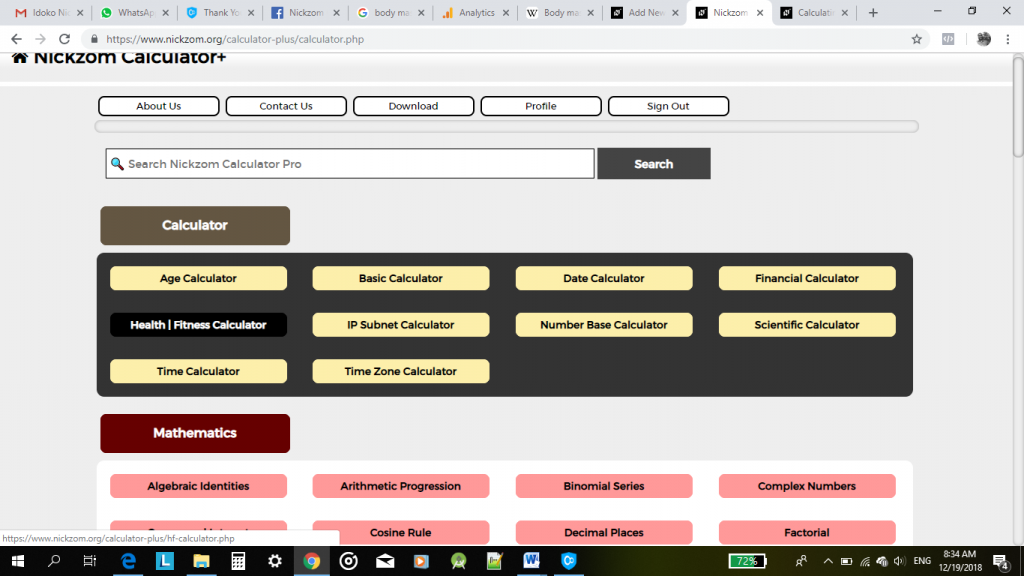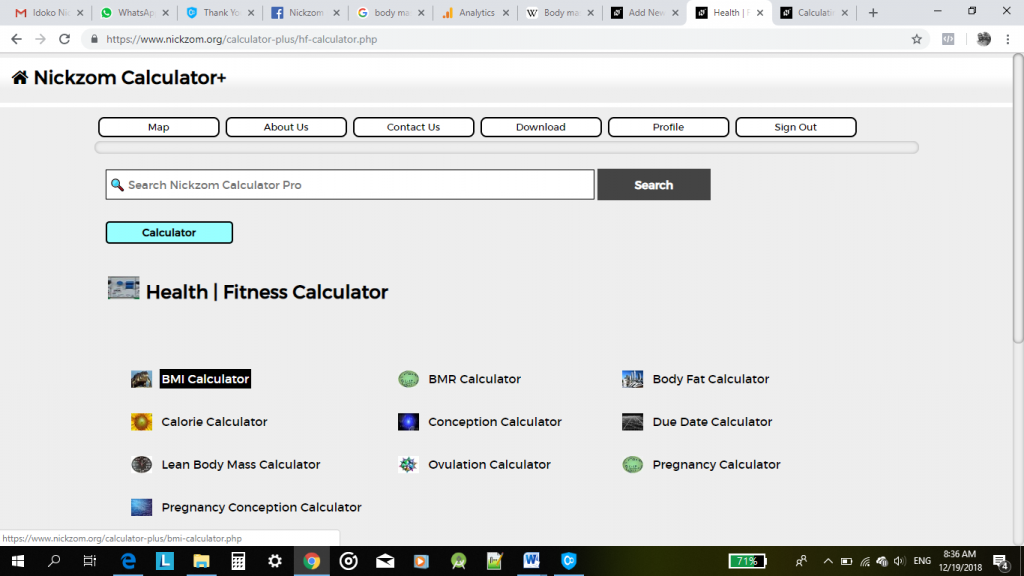## Nickzom Calculator Calculates Body Mass Index

According to Wikipedia,

The body mass index (BMI) or Quetelet index is a value derived from the mass (weight) and height of an individual. The BMI is defined as the body mass divided by the square of the body height, and is universally expressed in units of kg/m2, resulting from mass in kilograms and height in metres.

Nickzom Calculator is the very first calculator encyclopedia to come out from Nigeria. It is capable of solving over 50,000 calculations and presenting the steps (workings). Fortunately, Nickzom Calculator can solve for your body mass index.

You can access Nickzom Calculator via any of these means:
Web – https://www.nickzom.org/calculator-plus